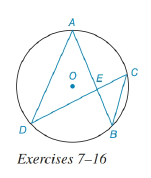Chapter 6.3, Problem 11E### Elementary Geometry for College St...

6th Edition
Daniel C. Alexander + 1 other
ISBN: 9781285195698

#### Solutions

Chapter
Section### Elementary Geometry for College St...

6th Edition
Daniel C. Alexander + 1 other
ISBN: 9781285195698
Textbook Problem
1 views

# In the figure for Exercises 7 to 16, O is the center of the circle. See Theorem 6.3.5.Given: A E = 6 , E C = 3 , A D = 8 Find: CB.To determine

To find:

To find CB.

Explanation

The diagrammatic representation is given below,

Theorems:

1. If two chords intersect within a circle, then the product of the lengths of the segments (parts) of one chord is equal to the product of the lengths of the segments of the other chord. Now compare the triangles ΔAED,ΔCEB

2. If two inscribed angles intercept the same arc, then these angles congruent. By using the above theorem mADE=mCBE. Vertical angles are congruent to each other. (The angles that are opposite to each other are vertical angles)

### Still sussing out bartleby?

Check out a sample textbook solution.

See a sample solution

#### The Solution to Your Study Problems

Bartleby provides explanations to thousands of textbook problems written by our experts, many with advanced degrees!

Get Started

#### (rn)4r52n

Applied Calculus for the Managerial, Life, and Social Sciences: A Brief Approach

#### Sketch the graph of y=x24.

Calculus: An Applied Approach (MindTap Course List)

#### True or False: The graph in question 3 has a vertical asymptote at x = 1.

Study Guide for Stewart's Single Variable Calculus: Early Transcendentals, 8th